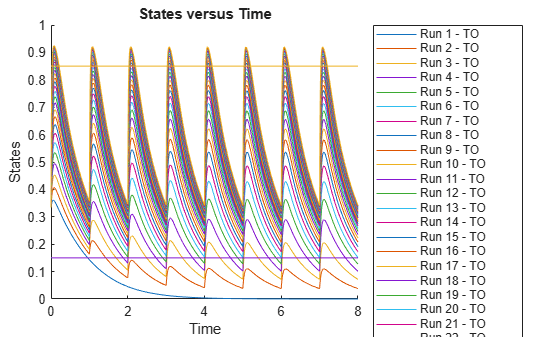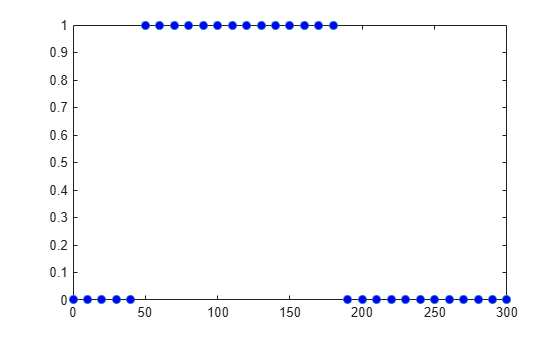# renameobservable

Rename observables in SimData

## Syntax

``sdout = renameobservable(sdin,oldNames,newNames)``

## Description

example

````sdout = renameobservable(sdin,oldNames,newNames)` returns a new `SimData` object (or array of objects) `sdout` after renaming observables in `sdin` and recalculating all observable expressions.```

## Examples

collapse all

`sbioloadproject tmdd_with_TO.sbproj`

Set the target occupancy (`TO`) as a response.

```cs = getconfigset(m1); cs.RuntimeOptions.StatesToLog = 'TO';```

Get the dosing information.

`d = getdose(m1,'Daily Dose');`

Scan over different dose amounts using a `SimBiology.Scenarios` object. To do so, first parameterize the `Amount` property of the dose. Then vary the corresponding parameter value using the `Scenarios` object.

```amountParam = addparameter(m1,'AmountParam','Units',d.AmountUnits); d.Amount = 'AmountParam'; d.Active = 1; doseSamples = SimBiology.Scenarios('AmountParam',linspace(0,300,31));```

Create a `SimFunction` to simulate the model. Set `TO `as the simulation output.

```% Suppress informational warnings that are issued during simulation. warning('off','SimBiology:SimFunction:DOSES_NOT_EMPTY'); f = createSimFunction(m1,doseSamples,'TO',d)```
```f = SimFunction Parameters: Name Value Type Units _______________ _____ _____________ ____________ {'AmountParam'} 1 {'parameter'} {'nanomole'} Observables: Name Type Units ______ _____________ _________________ {'TO'} {'parameter'} {'dimensionless'} Dosed: TargetName TargetDimension Amount AmountValue AmountUnits _______________ ___________________________________ _______________ ___________ ____________ {'Plasma.Drug'} {'Amount (e.g., mole or molecule)'} {'AmountParam'} 1 {'nanomole'} ```
`warning('on','SimBiology:SimFunction:DOSES_NOT_EMPTY');`

Simulate the model using the dose amounts generated by the `Scenarios` object. In this case, the object generates 31 different doses; hence the model is simulated 31 times and generates a `SimData` array.

```doseTable = getTable(d); sd = f(doseSamples,cs.StopTime,doseTable)```
``` SimBiology Simulation Data Array: 31-by-1 ModelName: TMDD Logged Data: Species: 0 Compartment: 0 Parameter: 1 Sensitivity: 0 Observable: 0 ```

Plot the simulation results. Also add two reference lines that represent the safety and efficacy thresholds for `TO`. In this example, suppose that any `TO` value above 0.85 is unsafe, and any `TO` value below 0.15 has no efficacy.

```h = sbioplot(sd); time = sd(1).Time; h.NextPlot = 'add'; safetyThreshold = plot(h,[min(time), max(time)],[0.85, 0.85],'DisplayName','Safety Threshold'); efficacyThreshold = plot(h,[min(time), max(time)],[0.15, 0.15],'DisplayName','Efficacy Threshold');```Postprocess the simulation results. Find out which dose amounts are effective, corresponding to the `TO` responses within the safety and efficacy thresholds. To do so, add an observable expression to the simulation data.

```% Suppress informational warnings that are issued during simulation. warning('off','SimBiology:sbservices:SB_DIMANALYSISNOTDONE_MATLABFCN_UCON'); newSD = addobservable(sd,'stat1','max(TO) < 0.85 & min(TO) > 0.15','Units','dimensionless')```
``` SimBiology Simulation Data Array: 31-by-1 ModelName: TMDD Logged Data: Species: 0 Compartment: 0 Parameter: 1 Sensitivity: 0 Observable: 1 ```

The addobservable function evaluates the new observable expression for each `SimData` in `sd` and returns the evaluated results as a new `SimData` array, `newSD`, which now has the added observable (`stat1`).

SimBiology stores the observable results in two different properties of a `SimData` object. If the results are scalar-valued, they are stored in `SimData.ScalarObservables`. Otherwise, they are stored in `SimData.VectorObservables`. In this example, the `stat1` observable expression is scalar-valued.

Extract the scalar observable values and plot them against the dose amounts.

```scalarObs = vertcat(newSD.ScalarObservables); doseAmounts = generate(doseSamples); figure plot(doseAmounts.AmountParam,scalarObs.stat1,'o','MarkerFaceColor','b')```The plot shows that dose amounts ranging from 50 to 180 nanomoles provide `TO` responses that lie within the target efficacy and safety thresholds.

You can update the observable expression with different threshold amounts. The function recalculates the expression and returns the results in a new `SimData` object array.

`newSD2 = updateobservable(newSD,'stat1','max(TO) < 0.75 & min(TO) > 0.30');`

Rename the observable expression. The function renames the observable, updates any expressions that reference the renamed observable (if applicable), and returns the results in a new `SimData` object array.

`newSD3 = renameobservable(newSD2,'stat1','EffectiveDose');`

Restore the warning settings.

`warning('on','SimBiology:sbservices:SB_DIMANALYSISNOTDONE_MATLABFCN_UCON');`

## Input Arguments

collapse all

Input simulation data, specified as a `SimData` object or array of objects.

Existing names of the observables, specified as a character vector, string, string vector, or cell array of character vectors.

Example: `{'max_drug','mean_drug'}`

Data Types: `char` | `string` | `cell`

New names for the observables, specified as a character vector, string, string vector, or cell array of character vectors. The number of new names must match the number of old names.

Each new name must be unique in the `SimData` object, meaning it cannot match the name of any other observable, species, compartment, parameter, or reaction referenced in the `SimData` object.

Example: `{'MAX','MEAN'}`

Data Types: `char` | `string` | `cell`

## Output Arguments

collapse all

Simulation data with observable results, returned as a `SimData` object or array of objects.